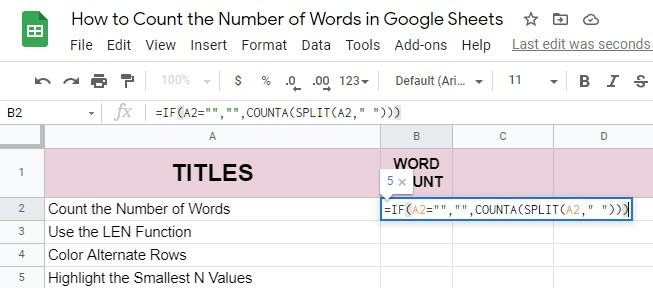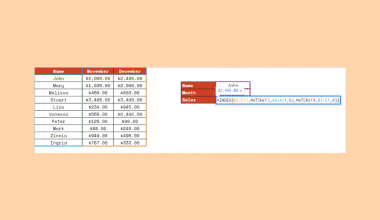# How to Count the Number of Words in Google Sheets

Knowing how to count the number of words in Google Sheets is useful if you need to know the exact number of words in a spreadsheet or in a specific cell, row, or column.

Even though Google Sheets is mostly used with numbers (while Google Docs is used for text), sometimes you’ll have a word count limit per cell or the formula you’ll use will require a number of words. If you’ve ever been in any similar situation, you’ve probably wondered if there’s an easy way to count the number of words in Google Sheets.

What you should know is that there’s no built-in feature (like in Google Docs) you can use. However, with a combination of a few functions, you can easily get the word count in your spreadsheet.

Depending on whether you just need the total number of words in Google Sheets or you’re actually looking for some variations, such as the total word count of an entire column/in a specific cell or the word count without specific words, there is more than one way to count the number of words. In this article, we’ll cover all of them so that you no longer have to spend time manually counting the number of words in your spreadsheets!

Since we have a lot to cover, let’s go straight to real examples where we’ll deal with actual values!

## How to Count the Number of Words in a Cell in Google Sheets (Using the LEN Function)

Say you have a sheet with the list of titles, and each of the titles cannot be longer than 5 words. Sure, you can manually count the words in each and every title but what if there are thousands of them?

Instead of manually counting the words, we can use the LEN function along with the SUBSTITUTE function, to count the number of spaces in between the words. If there’s one space, that will mean that there are two words, and if there are two spaces, that would mean that there are three words, and so on.

1. First, click on the cell where you want to input the total number of words. We’ll start with cell B2.1. Paste the =LEN(A2)-LEN(SUBSTITUTE(A2,” “,””))+1 formula and press ENTER on your keyboard to close the formula and output the result.1. In our example, the result in cell B2 should be 5.
2. Since we need to count the number of words in each cell in column A, select the cell B2 and drag its bottom right corner to input the same formula to other cells in column B. Google Sheets will automatically adjust the formula, so in cell B3 the formula will be =LEN(A3)-LEN(SUBSTITUTE(A3,” “,””))+1.Now, in column B we have a list of the number of words in matching cells in column A. However, we still don’t know which of these cells contains text with more than 5 words. To find out this, we can use conditional formatting.

1. Select column B and choose Format > Conditional Formatting from the menu. This will open the ‘Conditional format rules’ toolbar on the right.1. Change the range to B2:B1001, select ‘Format cells if greater than…’ from the format rules drop-down menu, and enter the value to the text box below. In our example, we need to highlight all the cells in column B where the result is greater than 5.1. You’ll see that the format rules will be applied automatically. Click on the ‘Done’ button below to save your conditional format rule, and close the toolbar by clicking the ‘X’ button in the upper right corner.

## How to Count the Number of Words in a Cell in Google Sheets (Using the COUNTA and SPLIT Functions)

There’s another formula that works on a similar principle. We can also use the COUNTA and SPLIT functions, which will separate the text in a string based on the delimiter (and in our example, the delimiter will be a space character).

1. Select the cell B2 and paste the following formula =IF(A2=””,””,COUNTA(SPLIT(A2,” “))).1. Press ENTER on your keyboard. The result in cell B2 will be 5. If Google Sheets suggest that you auto-fill the column with this formula, you should accept that, otherwise, do this by yourself.That’s it! Now, column B contains the word count of text strings from cells in column A. Apply the same conditional format rule as above, and you’ll easily find out what text strings in column A have more than 5 words.

You can also use the =COUNTA(SPLIT(A2,” “)) formula, but unlike the above formula, this one will return 1 as a result if the cell is empty (the formula shown above will leave the output cell empty).Since we have a lot to cover in this article, we won’t explain how each of these functions works. However, if you’re interested to find out more about their anatomy and how you can use them to create your own formulas, check out our articles about how to use the IF function, the anatomy of the COUNTA function, and how to use the SPLIT function in Google Sheets.

Please note that both these formulas work only if there are no extra spaces between the words. If there’s more than one space between two words, you’ll get the wrong result. Luckily, we have a solution for this problem! You can use the TRIM function. Instead of the formula we’ve used before, we would use =LEN(TRIM(A2))-LEN(SUBSTITUTE(TRIM(A2),” “,””))+1 or =IF(TRIM(A2)=””,””,COUNTA(SPLIT(TRIM(A2),” “))).## How to Count the Number of Words in an Entire Column in Google Sheets

If you need to count the number of words in an entire column in Google Sheets, you can use the above formulas along with the ARRAYFORMULA function we use when we want to apply a formula to an entire column.

The first formula, with the LEN function will now be =ARRAYFORMULA(SUM(LEN(range)-LEN(SUBSTITUTE(range,” “,””))+1)), while the other one will be =ARRAYFORMULA(SUM(COUNTA(SPLIT(range,” “)))). Please remember that both these two formulas will count the empty cells as 1.## How to Count the Number of Specific Words in a Cell in Google Sheets

Let’s say you don’t need the total word count but only to count the number of specific words in a cell in Google Sheets. You can do that by using the COUNTIF function. This function combines both the IF and COUNT function in one. To count the number of specific words in a cell, use the following formula =IF(cell=””,””,COUNTIF(SPLIT(cell,” “),”criterion”)) just change the ‘criterion’ with the specific word you want to count.## How to Count the Number of Words Without a Specific Word in a Cell in Google Sheets

You can use the same logic if you need to count the number of words without a specific word or string in a cell in Google Sheets. The formula we’ll use is =IF(cell=””,””,COUNTIF(SPLIT(cell,” “),”<>criterion”)).## How to Count the Number of Words of a Specific Length in a Cell in Google Sheets

If for any reason, you’d want to count the number of words of a specific length in a cell in Google Sheets, you can use the following formula =ARRAYFORMULA(COUNTIF(LEN(SPLIT(cell,” “)),”>1″)). This formula will count the number of words longer than 1 letter. To count the words longer than two letters, change 1 to 2, and so on.Now you know several ways to count the number of words in Google Sheets, depending on your criteria. Make a copy of our spreadsheet and try any or all of these formulas for yourself:

You can also take a look at our other Google Sheets formulas you can use to create various formulas that will help you create even more powerful formulas. 🙂Our goal this year is to create lots of rich, bite-sized tutorials for Google Sheets users like you. If you liked this one, you'll love what we are working on! Readers receive ✨ early access ✨ to new content. There will be no spam and you can unsubscribe at any time.

##### You May Also Like## How to Use TRIMMEAN Function in Google Sheets

The TRIMMEAN function in Google Sheets is used to calculate the mean of a dataset excluding some proportion…## How To Use INDEX and MATCH Together in Google Sheets

The INDEX and MATCH together in Google Sheets come useful if you want to perform lookups. Using the…## How to Use AVERAGEIF Function in Google Sheets

The AVERAGEIF function in Google Sheets is useful if you want to get the average of a row…## How to Convert Month Name to Number in Google Sheets

Knowing how to convert month name to number in Google Sheets is useful if you have your month…